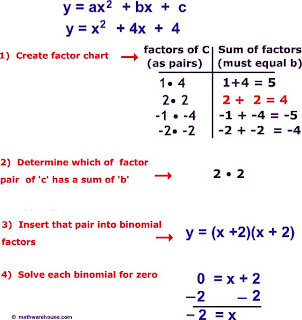Thursday, April 23, 2009

Revision

Exam is right around the corner. It's time to do revision on the topics that my students have learned so far. Topics that will be included in the exams are

1. Standard Form
2. Quadratic Expressions and Equation
3. Sets
4. The Straight Line

and some Form 3 topics. I will have to check with Mrs Chan on what those topics will be.

There's some good sites students can check on :

.
Below is some excerpt from one of the site

Example
Calculate the solutions of the quadratic equation below by factoring
quadratic equation: y = x² + 4x + 4

SolutionsYou can read more at the Math Warehouse site

The Straight Line
.

These links provide useful information about straight lines.

Interactive Mathematics

Maths Library
Thinkquest